## Projection methods in linear algebra numerics

Linear algebra classes often jump straight to the definition of a projector (as a matrix) when talking about orthogonal projections in linear spaces. As often as it happens, it is not clear how that definition arises. This is what is covered in this post.

## Orthogonal projection: how to build a projector

### Case 1 – 2D projection over (1,0)

It is quite straightforward to understand that orthogonal projection over (1,0) can be practically achieved by zeroing out the second component of any 2D vector, at last if the vector is expressed with respect to the canonical basis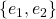. Albeit an idiotic statement, it is worth restating: the orthogonal projection of a 2D vector amounts to its first component alone.

How can this be put math-wise? Since we know that the dot product evaluates the similarity between two vectors, we can use that to extract the first component of a vector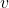. Once we have the magnitude of the first component, we only need to multiply that by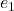itself, to know how much in the direction ofwe need to go. For example, starting from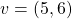, first we get the first component as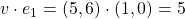; then we multiply this value by e_1 itself: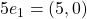. This is in fact the orthogonal projection of the original vector. Writing down the operations we did in sequence, with proper transposing, we get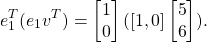One simple and yet useful fact is that when we project a vector, its norm must not increase. This should be intuitive: the projection process either takes information away from a vector (as in the case above), or rephrases what is already there. In any way, it certainly does not add any. We may rephrase our opening fact with the following proposition:

PROP 1: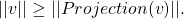This is can easily be seen through the pitagorean theorem (and in fact only holds for orthogonal projection, not oblique):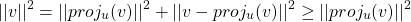## Reproducing a transport instability in convection-diffusion equation

Drawing from Larson-Bengzon FEM book, I wanted to experiment with transport instabilities. It looks there might be an instability in my ocean-ice model but before being able to address that, I wanted to wrap my head around the 1D simplest example one could find. And that is the Convection-Diffusion Equation:

(1)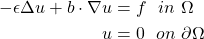The first term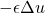is responsible of the smearing of the velocity field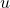proportionally to, and is thus called the diffusion term. Intuitively, it controls how much the neighbors of a given pointare influenced by the behavior of; how much velocities (or temperatures: think of whatever you want!) diffuse in the domain.

The second term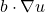controls how much the velocitiesare transported in the direction of the vector field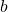, and is thus called the convective term. A requirement for this problem to be well-posed is that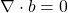— otherwise it would mean that we allow velocities to vanish or to come into existence.

The instability is particularly likely to happen close to a boundary where a Dirichlet boundary condition is enforced. The problem is not localized only close to the boundary though, as fluctuations can travel throughout the whole domain and lead the whole solution astray.

## Transport instability in 1D

The simplest convection-diffusion equation in 1D has the following form:

(2)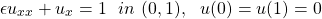whose solution, for smallis approximately just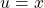. This goes well with the boundary condition at 0, but not with the condition at 1, where the solution needs to go down to 0 quite abruptly to satisfy the boundary condition.

It’s easy to simulate the scenario with FEniCS and get this result (with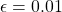and the unit interval divided in 10 nodes):in which we can infer two different trends: one with odd points and one with even points! In fact, if we discretize the 1D equation with finite elements we obtain:

(3)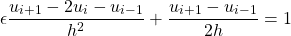## How do Dirichlet and Neumann boundary conditions affect Finite Element Methods variational formulations?

To solve a classical second-order differential problem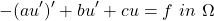with FEM, we first need to derive its weak formulation. This is achieved by multiplying the equation by a test function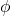and then integrating by parts to get rid of second order derivatives:

(1)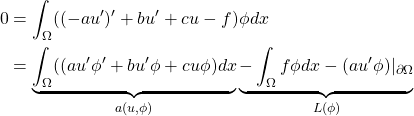A typical FEM problem then reads like: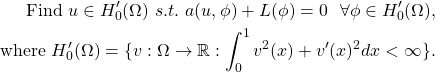What is the difference between imposing Dirichlet boundary conditions (ex.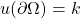) and Neumann ones (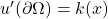) from a math perspective? Dirichlet conditions go into the definition of the space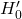, while Neumann conditions do not. Neumann conditions only affect the variational problem formulation straight away.

## What is the difference between Finite Differences and Finite Element Methods?

With Finite Differences, we discretize space (i.e. we put  a grid on it) and we seek the values of the solution function at the mesh points. We still solve a discretized differential problem.

With Finite Elements, we approximate the solution as a (finite) sum of functions defined on the discretized space. These functions make up a basis of the space, and the most commonly used are the hat functions. We end up with a linear system whose unknowns are the weights associated with each of the basis functions: i.e., how much does each basis function count for out particular solution to our particular problem?

Brutally, it is finding the value of the solution function at grid points (finite differences) vs the weight of the linear combinations of the hat functions (finite elements).